# Basics of paleoTS

## Introduction

The paleoTS package contains functions for analyzing paleontological time-series of trait data. It implements a set of evolutionary models that have been suggested to be important for this kind of data. These include simple models - those for which evolutionary dynamics do not change over the observed interval:

• Random walks, both unbiased (URW) and directional/biased versions (GRW)
• Stasis, modeled here as uncorrelated variation around a constant mean, along with and a strict version (StrictStasis) for when there is no real evolutionary change
• Ornstein-Uhlenbeck (OU) processes, which can be used to model microevolutionary adaptation to a local peak on the adaptive landscape
• covariate-tracking models (covTrack), in which changes in a trait follow changes in an external variable (e.g., body size evolves in response to temperature changes)

In addition, there are complex models that show heterogeneous evolutionary dynamics:

• rapid punctuations
• punctuations that are protracted enough to be sampled, modeled as stasis - general random walk - stasis
• mode shifts, for sequences that start in stasis and shift to a random walk, or vice versa

Models in paleoTS are fit via maximum-likelihood, using numerical optimization to find the best supported set of parameter values. Functions allow users to fit and compare models, plot data and model fits, and perform additional analyses.

## Getting data into paleoTS

The easiest way to import your own data into paleoTS is through the read.paleoTS function, which reads a text file with four columns corresponding to the means, variances, sample sizes, and ages of samples populations (in that order). This function converts the input into an object of class paleoTS that is the required input for most functions. See that function’s help page for options and more information.

Here, we’ll generate a time-series by simulation, and then plot it:

library(paleoTS)
set.seed(10)  # to make example replicatable
x <- sim.GRW(ns = 20, ms = 0.5, vs = 0.1)
plot(x)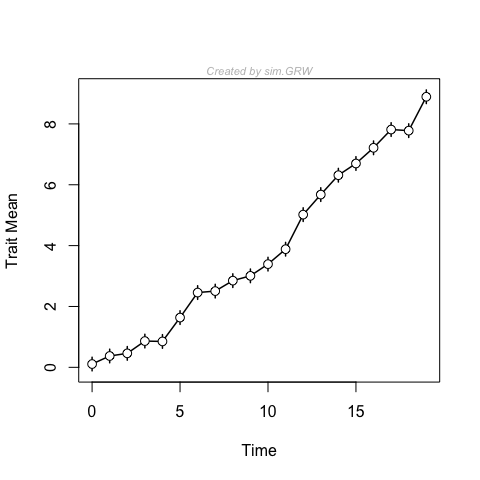There are simulation functions for all models that can be fit. You can look at x and see all the components of paleoTS objects:

print(str(x))
#> List of 9
#>  $mm : num [1:20] 0.108 0.373 0.459 0.863 0.851 ... #>$ vv       : num [1:20] 1 1 1 1 1 1 1 1 1 1 ...
#>  $nn : num [1:20] 20 20 20 20 20 20 20 20 20 20 ... #>$ tt       : int [1:20] 0 1 2 3 4 5 6 7 8 9 ...
#>  $MM : num [1:20] 0 0.506 0.948 1.014 1.325 ... #>$ genpars  : Named num [1:2] 0.5 0.1
#>   ..- attr(*, "names")= chr [1:2] "mstep" "vstep"
#>  $label : chr "Created by sim.GRW" #>$ start.age: NULL
#>  $timeDir : chr "increasing" #> - attr(*, "class")= chr "paleoTS" #> NULL Users will not generally modify any of this directly; rather this information is used and modified by paleoTS functions. For example, one can use the sub.paleoTS function to subset paleoTS objects: x.sub <- sub.paleoTS(x, ok = 10:15) # select only populations 10 through 15 plot(x) plot(x.sub, add = TRUE, col = "red")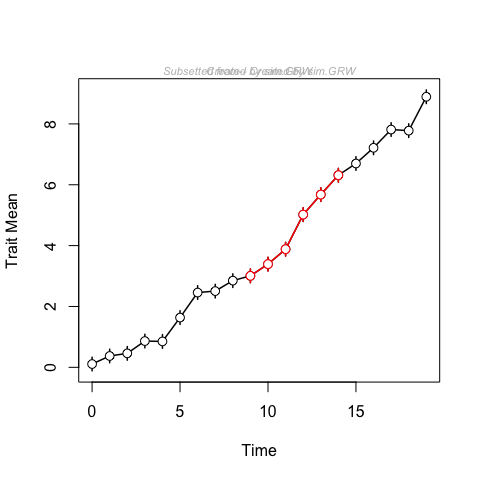### Fitting models The most common goal for users of paleoTS will be to fit and compare models. Simple models can be fit using the fitSimple function:  library(mnormt) # should omit this later w.grw <- fitSimple(x, model = "GRW") print(w.grw$parameters)  # look at estimated parameters
#>         anc       mstep       vstep
#> -0.02171219  0.45961231  0.05507781

The maximum-likelihood parameter estimates are reasonably close to the generating parameters. We can also visualize this model fit by plotting the data with 95% probability intervals for the model:

plot(x, modelFit = w.grw)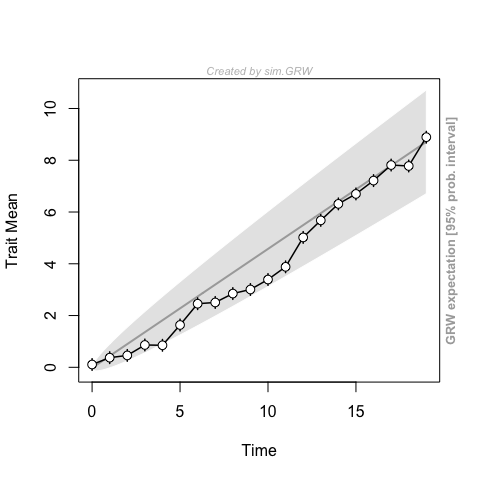In addition to interpreting parameter estimates, we often want to compare the fit of different models to the same data:

 w.urw <- fitSimple(x, model = "URW")
compareModels(w.grw, w.urw)  # convenient table comparing model support
#>
#> Comparing 2 models [n = 20, method = Joint]
#>
#>           logL K     AICc    dAICc Akaike.wt
#> GRW  -6.114511 3 19.72902  0.00000         1
#> URW -17.194526 2 39.09494 19.36591         0

Probably not surprisingly, model support is much higher for the GRW model than for the URW model. General random walks allow for directional evolution, whereas unbiased random walks do not.

Next, we’ll fit a model with punctuated changes, where the timing of the punctuations is not specified by the user. All possible shift points are tested (subject to the constraint that each segment has at least minb populations) and the best supported shift point is returned:

 w.punc <- fitGpunc(x, ng = 2)  # ng is the number of segments (= number of punctuations + 1)
#> Total # hypotheses:  7
#> 1  2  3  4  5  6  7

Visually, the fit of the punctuated model is not very compelling,

 plot(x, modelFit = w.punc)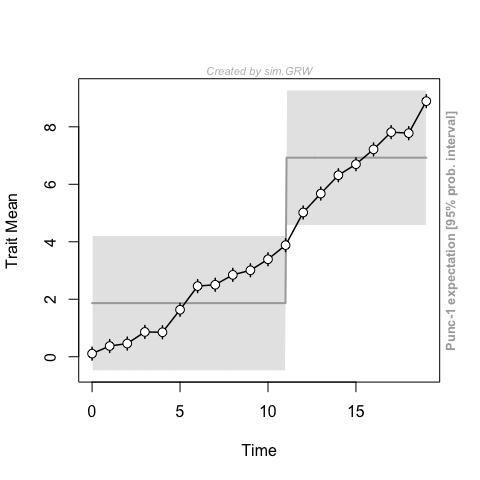and this model fares poorly compared the GRW model:

 compareModels(w.grw, w.urw, w.punc)
#>
#> Comparing 3 models [n = 20, method = Joint]
#>
#>              logL K     AICc    dAICc Akaike.wt
#> GRW     -6.114511 3 19.72902  0.00000         1
#> URW    -17.194526 2 39.09494 19.36591         0
#> Punc-1 -32.399374 4 75.46541 55.73639         0

Note that compareModels can take any number of model fits as arguments. Certain models are of general enough interest that paleoTS has convenience functions for fitting them:

 fit3models(x)
#>
#> Comparing 3 models [n = 20, method = Joint]
#>
#>              logL K      AICc    dAICc Akaike.wt
#> GRW     -6.114511 3  19.72902  0.00000         1
#> URW    -17.194526 2  39.09494 19.36591         0
#> Stasis -48.715248 2 102.13638 82.40736         0

This function fits the three most canonical models in the paleo literature: general random walk (direction evolution), unbiased random walk, and stasis.

## An Empirical Example: Bell’s stickleback data

Hunt et al. (2008) re-analyzed data Bell’s (year) capturing three traits in a highly resolved sequence of fossil sticklebacks from an ancient lake. These observation capture an initially highly armored species invading the lake. The species becomes less armored over time, initially quickly but then at a decelerating rate. The pattern is similar to the expected exponential approach to a new optimum expected when a population climbs a peak in the adaptive landscape via an OU process.

In the code below, we first omit levels with no measurable fossils, and then those before the invasion of the new species, so as to focus on the adaptive trajectory afterwards. Some of the samples have very low N, and so their variances are estimated imprecisely. We use the function to replace the variances in these low N samples with the estimated pooled variance (average variance, weighted by sample size).

data(dorsal.spines)
ok1 <- dorsal.spines$nn > 0 # levels without measured fossils ok2 <- dorsal.spines$tt > 4.4  # levels before the new species invades
ds.sub <- sub.paleoTS(dorsal.spines, ok = ok1 & ok2, reset.time = TRUE)  # subsample
ds.sub.pool <- pool.var(ds.sub, minN = 5, ret.paleoTS = TRUE)  # replace some pooled variance
w.ou <- fitSimple(ds.sub.pool, pool = FALSE, model = "OU")
plot(ds.sub.pool, modelFit = w.ou)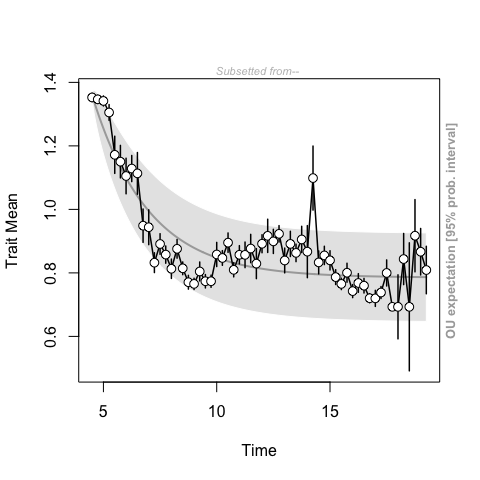## Joint versus AD parameterization

Nearly all models can be fit by two different methods or parameterizations, referred in paleoTS as Joint or AD, that reflect different strategies for handling temporal autocorrelation that is predicted by most models of trait evolution. The AD approach uses differences between adjacent points, which are expected to be independent, with the total log-likelihood being the sum of the individual log-likelihoods of the differences. It is a REML (restricted maximum-likelihood) approach, like phylogenetic independent contrasts. The Joint method is a full likelihood approach, with calculations based on the joint distribution of all populations in a sample. Under all models considered here, this joint distribution is multivariate normal. The Joint approach is the default throughout paleoTS.

The two approaches tend to produce similar parameter estimates and relative model fits under most circumstances. One situation in which the Joint approach is clearly better is that of a long, noisy trend, as shown here:

set.seed(90)
y <- sim.GRW(ns = 40, ms = 0.2, vs = 0.1, vp = 4)  # high vp gives broader error bars
plot(y)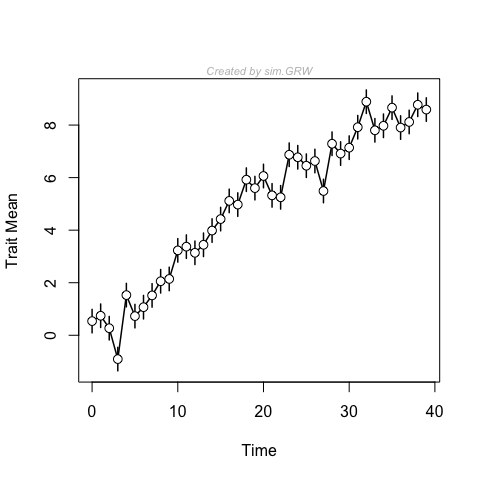fit3models(y, method = "Joint")  # GRW clearly wins
#>
#> Comparing 3 models [n = 40, method = Joint]
#>
#>             logL K      AICc      dAICc Akaike.wt
#> GRW    -38.68156 3  84.02978   0.000000     0.986
#> URW    -44.12027 2  92.56487   8.535092     0.014
#> Stasis -97.68580 2 199.69593 115.666150     0.000
fit3models(y, method = "AD")     # GRW only barely beats URW
#>
#> Comparing 3 models [n = 39, method = AD]
#>
#>             logL K      AICc      dAICc Akaike.wt
#> GRW    -45.11812 2  94.56958  0.0000000      0.56
#> URW    -46.47330 1  95.05471  0.4851313      0.44
#> Stasis -94.44239 2 193.21812 98.6485385      0.00